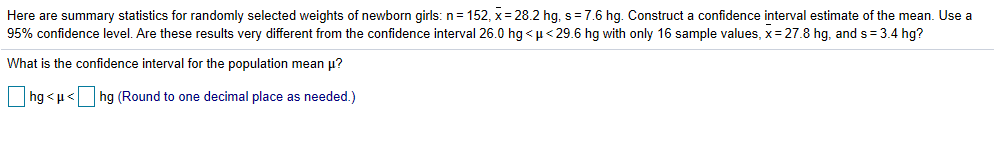# Here are summary statistics for randomly selected weights of newborn girls: n: 152, x-28.2 hg. s-76 hg. Construct a confidence interval estimate of the mean. Use a 95% confidence level. Are these results very different from the confidence interval 26.0 hg < μ < 296 hg ith only 16 sample values, x-278 hg, and s-3.4 hg? What is the confidence interval for the population mean μ? hg < μ< | | hg (Round to one decimal place as needed )

Questionhelp_outlineImage TranscriptioncloseHere are summary statistics for randomly selected weights of newborn girls: n: 152, x-28.2 hg. s-76 hg. Construct a confidence interval estimate of the mean. Use a 95% confidence level. Are these results very different from the confidence interval 26.0 hg < μ < 296 hg ith only 16 sample values, x-278 hg, and s-3.4 hg? What is the confidence interval for the population mean μ? hg < μ< | | hg (Round to one decimal place as needed ) fullscreen

3 Ratings

### Want to see the step-by-step answer?